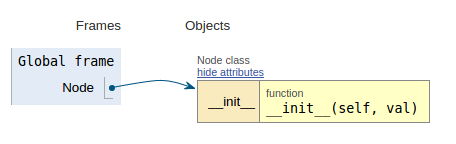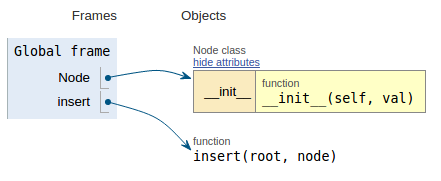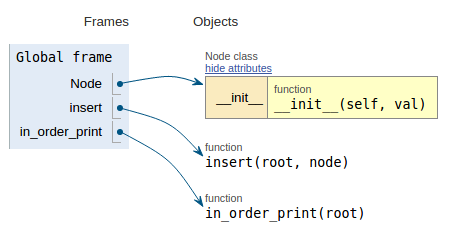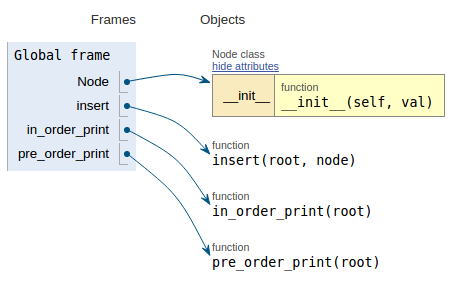# Binary Search Tree - Insertion (Python)

suggest change

This is a simple implementation of Binary Search Tree Insertion using Python.

An example is shown below:Following the code snippet each image shows the execution visualization which makes it easier to visualize how this code works.

``````class Node:
def __init__(self, val):
self.l_child = None
self.r_child = None
self.data = val````````````def insert(root, node):
if root is None:
root = node
else:
if root.data > node.data:
if root.l_child is None:
root.l_child = node
else:
insert(root.l_child, node)
else:
if root.r_child is None:
root.r_child = node
else:
insert(root.r_child, node)````````````def in_order_print(root):
if not root:
return
in_order_print(root.l_child)
print root.data
in_order_print(root.r_child)````````````def pre_order_print(root):
if not root:
return
print root.data
pre_order_print(root.l_child)
pre_order_print(root.r_child)``````